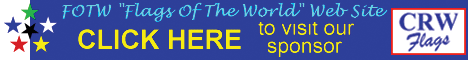# Bibliography: Authorship index: sorted by surname (A)

## Cross-index page: under construction!

Keywords: (no keywords) |
Links: FOTW homepage | search | disclaimer and copyright | write us | mirrors

Contents:

## Latin script

### A

• [ism] Aʔsmaʕil ‭(A.)‬
• [aab] Aaby ‭(G.)‬
• [agd] Aagedal ‭(O.)‬
• [jaa] Aall ‭(L.)‬
• [aar] Aarnes ‭(S.)‬
• [aiv] Aars ‭(I.)‬
• [aas] Aas
• [brz] AB
• [abz] Abaza ‭(V.)‬
• [abb] Abbe ‭(C.)‬
• [whb] Abdel-Wahab ‭(S)‬
• [abl] Abeles ‭(M.)‬
• [abs] Abelson ‭(N.)‬
• [abj] Abér ‭(J.)‬
• [abe] Åberg ‭(A.)‬
• [abi] Abia ‭(J.)‬
• [brz] abr
• [abu] Abukhanfusa ‭(K.)‬
• [aco] Achen ‭(C.)‬
• [ach] Achen ‭(S.)‬
• [hja] Achermann ‭(H.)‬
• [aoy] Ackroyd ‭(P.)‬
• [ugg] af Ugglas ‭(C.)‬
• [jkl] Agnar Kl. J.
• [agw] Agnew ‭(C.)‬
• [yax] Âgnịxak ‭(V.)‬
• [ape] Aguiar ‭(P.)‬
• [agr] Aguirre ‭(M.)‬
• [agu] Agulhon ‭(M.)‬
• [ahr] Ahrenberg ‭(J.)‬
• [ahn] Ahrens ‭(H.)‬
• [aik] Aikman ‭(L.)‬
• [akj] Aikojen ‭(K.)‬
• [ail] Ailes ‭(A.)‬
• [ais] Aisantiea ‭(G.)‬
• [avz] Aivazyan ‭(S.)‬
• [ajz] Ajnenkiel ‭(A.)‬
• [yak] Âkimoviĉ ‭(B.)‬
• [aks] Akselson ‭(E.)‬
• [ala] Alardo ‭(C.)‬
• [alw] Alban ‭(L.)‬
• [abt] Alberti ‭(F.)‬
• [abk] Albinsky ‭(H.)‬
• [eff] Albrecht
• [aji] Albrechtová ‭(J.)‬
• [ato] Alden ‭(T.)‬
• [roo] Alderwerelt ‭(J.)‬
• [agt] Aldrighetti ‭(G.)‬
• [alf] Alef ‭(G.)‬
• [ald] Alegría ‭(J.)‬
• [als] Ales ‭(S.)‬
• [ale] Alexander ‭(K.)‬
• [axa] Alexandri ‭(A.)‬
• [zax] Alexy ‭(Z.)‬
• [afl] Alföldi ‭(A.)‬
• [ala] Allard ‭(C.)‬
• [aln] Allen ‭(M.)‬
• [aro] Allen ‭(R.)‬
• [dle] Allessandro ‭(R.)‬
• [alb] Allmayer-Beck ‭(J.)‬
• [all] Allport ‭(R.)‬
• [alm] Almgren ‭(C.)‬
• [ags] Alonso ‭(J.)‬
• [alg] Alt ‭(G.)‬
• [alt] Alter ‭(P.)‬
• [avv] Álvarez ‭(J.)‬
• [faz] Álvarez ‭(M.el)‬
• [azr] Álvarez ‭(M.ª)‬
• [alv] Álvarez ‭(Ra.)‬
• [azm] Álvarez ‭(Ru.)‬
• [amn] Amann ‭(T.)‬
• [aml] Amaral ‭(M.)‬
• [azi] Amerlinck ‭(T.)‬
• [ame] Ames
• [amz] Amézcua ‭(M.)‬
• [amo] Amón ‭(S.)‬
• [atl] Amstler ‭(J.)‬
• [atz] Amstutz ‭(W.)‬
• [amu] Amundsen ‭(B.)‬
• [asr] Amundsen ‭(S.)‬
• [amy] Amy ‭(R.)‬
• [aaw] Andersen ‭(A.)‬
• [ajw] Anderson ‭(J.)‬
• [ans] Anderson ‭(S.)‬
• [ank] Andolenko ‭(S.)‬
• [ane] Andreae ‭(B.)‬
• [asj] Andreassen ‭(J.)‬
• [oaw] Andrew ‭(O.)‬
• [arj] Andrić ‭(Đ.)‬
• [arj] Andrịĉ ‭(Dẑ.)‬
• [anm] Andrić ‭(M.)‬
• [ata] Andrieş-Tabac ‭(S.)‬
• [arj] Andrit̂ ‭(D̂.)‬
• [agl] Angell ‭(S.)‬
• [ang] Angst ‭(W.)‬
• [acj] Anker ‭(C.)‬
• [akh] Ankershofen ‭(G.)‬
• [aof] Ansoff ‭(P.)‬
• [ant] Antonetti ‭(P.)‬
• [ynu] Ânuŝkeviĉ ‭(I.)‬
• [apa] Aparicio ‭(J.)‬
• [app] Appelt ‭(H.)‬
• [apl] Appleton ‭(D.)‬
• [apu] Appuhn ‭(H.)‬
• [abr] Arbel ‭(R.)‬
• [acz] Arcarazo ‭(L.)‬
• [arc] Arce ‭(L.)‬
• [acx] Arceneaux ‭(T.)‬
• [ajq] Archambault ‭(J.)‬
• [abd] Archbold ‭(R.)‬
• [aga] Arco ‭(F.)‬
• [pda] Arcos ‭(I.)‬
• [agn] Arendar ‭(G.)‬
• [arg] Arge ‭(N.)‬
• [ahm] Arheim ‭(T.)‬
• [arp] Arias ‭(L.)‬
• [ark] Arkell ‭(A.)‬
• [amg] Armengol ‭(L.)‬
• [ams] Armstrong ‭(E.)‬
• [arm] Armstrong ‭(R.)‬
• [aru] Arnau ‭(R.)‬
• [arn] Arndt ‭(J.)‬
• [abv] Arnold ‭(B.)‬
• [yao] Ârova ‭(E.)‬
• [yao] Ârova ‭(O.)‬
• [azq] Arredondo ‭(E.)‬
• [arr] Arrigoni ‭(M.)‬
• [aak] Arstal ‭(A.)‬
• [art] Artamonov ‭(V.)‬
• [anj] Artelina
• [arv] Artimovich ‭(N.)‬
• [atu] Artusi ‭(L.)‬
• [avc] Arvidsson ‭(C.)‬
• [ase] Asensi ‭(F.)‬
• [asp] Ashley ‭(H.)‬
• [ash] Ashley ‭(L.)‬
• [apt] Asperts ‭(R.)‬
• [asn] Aspinall ‭(R.)‬
• [atg] Atlagić ‭(M.)‬
• [atg] Atlagid̂ ‭(М.)‬
• [aub] Aubert ‭(L.)‬
• [aud] Audin ‭(A.)‬
• [auf] Aufrère ‭(G.)‬
• [aue] Augé ‭(P.)‬
• [aug] Augustin ‭(J.)‬
• [avd] Avdẹẹv ‭(A.)‬
• [avl] Avellino ‭(L.)‬
• [avi] Avignone ‭(A.)‬
• [vaw] Awouters ‭(L.)‬
• [aya] Ayala ‭(L.)‬
• [avz] Ayvazyan ‭(S.)‬
• [azv] Azevedo ‭(F.)‬
• [azv] Azevedo ‭(S.)‬